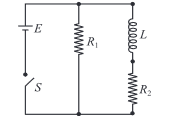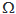# An inductor of inductance L = 400 mH and resistors of resistances R1 = 2  and R2 = 2  are connected to a battery of emf 12 V...An inductor of inductance L = 400 mH and resistors of resistances R1 = 2and R2 = 2are connected to a battery of emf 12 V as shown in the figure. The internal resistance of the battery is negligible. The switch S is closed at t = 0. What will be the potential drop across L as a function of time?

Anonymous User Physics Electromagnetic Induction & Alternating Current 09 May, 2020 65 views